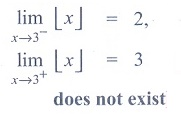# Chapter 9: Differential Calculus Limits and Continuity - Online Test

Q1.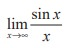Explaination / Solution:Q2.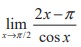Explaination / Solution: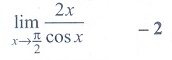Q3.Explaination / Solution:
No Explaination.

Q4.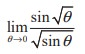Explaination / Solution: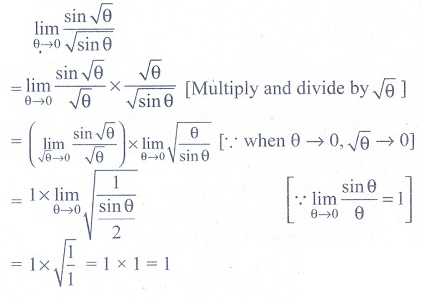Q5.is
Explaination / Solution:
No Explaination.

Q6.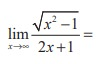Explaination / Solution: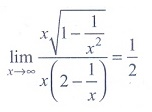Q7.Explaination / Solution:
No Explaination.

Q8.Explaination / Solution:
No Explaination.

Q9. If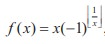, then the value of limx0 f (x) is equal to
Explaination / Solution:
No Explaination.

Q10.Explaination / Solution: